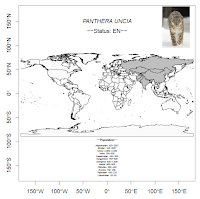## 29 Jun 2012

### Use IUCN API with R & XPathThanks to a posting on R-sig-eco mailing list I learned of the IUCN-API. Here's a simple example for what can be done with it (output as pdf is HERE):

## 25 Jun 2012

### Avoid Overplotting of Text in Ordination Diagram

Referring to a recent posting on r-sig-eco mailing list I'll add this example to theBioBucket:

```library(vegan)
library(vegan)
data(dune)
sol <- metaMDS(dune)

# use ordipointlabel -
# here is an example where I added cex according to species frequencies:
plot(sol, type = "n")
cex.lab = colSums(dune > 0) / nrow(dune) + 1
col.lab = rgb(0.2, 0.5, 0.4, alpha = 0.6)
ordipointlabel(sol, displ = "sp", col = col.lab, cex = cex.lab)

# you could also use pointLabel() from maptools package:
library(maptools)
x = as.vector(sol\$species[,1])
y = as.vector(sol\$species[,2])
w = row.names(sol\$species)

plot(sol, type = "n")
points(sol, displ = "species", cex = 1, pch = 4, col = 3)
pointLabel(x, y, w, col = col.lab, cex = cex.lab)```